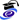# Sisto BaldoPosition
Associate Professor
MAT/05 - MATHEMATICAL ANALYSIS
Research sector (ERC)
PE1_8 - Analysis

Office
Ca' Vignal 2,  Floor 2,  Room 8B
Telephone
045 802 7935
Fax
045 802 7068
E-mail
sistobaldounivritPersonal web page
http://profs.sci.univr.it/~baldo

#### Office Hours

Monday, Hours 1:00 PM - 3:00 PM,   Ca' Vignal 2, Floor 2, room 8B

Curriculum
•Curriculum_ENG   (pdf, it, 46 KB, 18/10/17)
•Curriculum_ITA   (pdf, it, 66 KB, 18/10/17)

Sisto Baldo si occupa di Analisi Matematica.
I suoi interessi di ricerca vertono principalmente su Calcolo delle Variazioni, Teoria Geometrica della Misura, Equazioni alle Derivate Parziali e Problemi Variazionali Geometrici.
Si occupa anche di didattica della matematica, di formazione insegnanti  e di comunicazione informale della matematica per un pubblico generale.

#### Modules

Modules running in the period selected: 42.
Click on the module to see the timetable and course details.

Course Name Total credits Online Teacher credits Modules offered by this teacher
Master's degree in Mathematics Functional analysis (2019/2020)   12Bachelor's degree in Applied Mathematics Mathematical analysis 1 (2019/2020)   12Bachelor's degree in Applied Mathematics Mathematical analysis 2 (2019/2020)   12Master's degree in Mathematics Functional analysis (2018/2019)   12(Teoria 1)
Bachelor's degree in Applied Mathematics Mathematical analysis 1 (2018/2019)   12Bachelor's degree in Applied Mathematics Mathematical analysis 2 (2018/2019)   12(Teoria 2)
Master's degree in Mathematics Functional analysis (2017/2018)   12Bachelor's degree in Applied Mathematics Mathematical analysis 1 (2017/2018)   12Bachelor's degree in Applied Mathematics Mathematical analysis 2 (2017/2018)   12(Teoria 1)
Master's degree in Mathematics Functional analysis (2016/2017)   12Bachelor's degree in Applied Mathematics Mathematical analysis 1 (2016/2017)   12Bachelor's degree in Applied Mathematics Mathematical analysis 2 (2016/2017)   12(Teoria 1)
Master's degree in Mathematics Functional analysis (2015/2016)   12    (Parte 1)
Bachelor's degree in Applied Mathematics Mathematical analysis 1 (2015/2016)   12    (teoria)
Bachelor's degree in Applied Mathematics Mathematical analysis 2 (2015/2016)   12    (teoria)
Master's degree in Mathematics Functional analysis (2014/2015)   12    (Parte 1)
Bachelor's degree in Applied Mathematics Mathematical analysis 1 (2014/2015)   12    (Teoria)
Bachelor's degree in Applied Mathematics Mathematical analysis 2 (2014/2015)   12    (Teoria)
TFA A059 - Mathematics, chemistry, physics and natural sciences for middle school Didattica della matematica (2014/2015)   6DIDATTICA DELLA MATEMATICA 2
PAS A059 Mathematics and science in middle schools Matematica e didattica della matematica (2014/2015)   8MODULO B (e-learning)
MODULO B (teoria)
Master's degree in Mathematics Functional analysis (2013/2014)   12    (Parte 1)
Bachelor's degree in Applied Mathematics Mathematical analysis 1 (2013/2014)   12    (Teoria)
Bachelor's degree in Applied Mathematics Mathematical analysis 2 (2013/2014)   12    (Teoria 1)
PAS A059 Mathematics and science in middle schools Matematica e didattica della matematica (2013/2014)   8MODULO B (Teoria)
MODULO B (E-learning)
Master's degree in Mathematics Functional analysis (2012/2013)   12    (Teoria)
Bachelor's degree in Applied Mathematics Mathematical analysis 1 (2012/2013)   12    (Teoria)
Bachelor's degree in Applied Mathematics Mathematical analysis 2 (2012/2013)   12    (Teoria)
Master's degree in Mathematics Functional anaysis (2011/2012)   12    (Teoria)
Bachelor's degree in Applied Mathematics Mathematical analysis 1 (2011/2012)   12    (Teoria)
Bachelor's degree in Applied Mathematics Mathematical analysis 2 (2011/2012)   12    (Teoria)
Master's degree in Mathematics Functional anaysis (2010/2011)   12    (Esercitazioni)
(Teoria)
Bachelor's degree in Applied Mathematics Mathematical analysis 1 (2010/2011)   12    (Teoria)
Bachelor's degree in Applied Mathematics Mathematical analysis 2 (2010/2011)   12    (Teoria)
Master's degree in Mathematics Functional anaysis (2009/2010)   12    (Teoria)
Bachelor's degree in Applied Mathematics Mathematical analysis 1 (2009/2010)   12    (Teoria)
Bachelor's degree in Applied Mathematics Mathematical analysis 2 (2009/2010)   12    (Teoria)
Bachelor's degree in Bioinformatics (until 2008-2009) Mathematical analysis 1 (2008/2009)   6    mod.1
mod.2
Degree in Applied Mathematics (until a.y. 2008/2009) Mathematical analysis 1 (2008/2009)   9    mod.1

#### Research groups

Calculus of Variations and PDE
Il gruppo si occupa di attività di ricerca nel campo del calcolo delle variazioni, teoria geometrica della misura, teoria del controllo ottimo, teoria del trasporto ottimo, e applicazioni.
Mathematics - applications and modelling
Skills
Topic Description Research area
Existence theories Minimal surfaces. Calculus of variations on manifolds. Mathematics - applications and modelling
Calculus of variations and optimal control; optimization
Manifolds Geometric variational and evolution problems: minimal surfaces, motion by mean curvature. Optimal mass transport theory. Mathematics - applications and modelling
Calculus of variations and optimal control; optimization
Optimality conditions. Asymptotics of variational problems. Variational convergences and Gamma Convergence. Singular perturbations of variational problems. Mathematics - applications and modelling
Calculus of variations and optimal control; optimization
Variational principles of physics. Variational problems from condensed matter and particle Physics (e.g. Ginzburg-Landau models for superconductivity, Gross-Pitaevskii model for Bose-Einstein condensation, string theory) and their relation with minimal surfaces. Mathematics - applications and modelling
Calculus of variations and optimal control; optimization
Projects
Title Starting date
Trasporto ottimo di massa, disuguaglianze geometriche e funzionali e applicazioni (PRIN 2008 ESTERNO) 3/22/10
Metodi variazionali nella teoria del trasporto ottimo di massa e nella teoria geometrica della misura (PRIN 2006 ESTERNO) 2/9/07
Calcolo delle Variazioni (PRIN 2004 ESTERNO) 11/30/04
Calcolo delle Variazioni (PRIN 2002 ESTERNO) 12/16/02